# 4. Vector dynamics

## IntroductionIsaac Newton had a very ingenious idea when he was 23, which would later develop into his famous theory of gravitation and the laws of motion. Newton thought that in the same way as an apple falls to the Earth, due to its gravitational attraction, so the Moon is also falling to the Earth under the same gravitational attraction of the Earth. The reason why the Moon never reaches the surface of the Earth, as the apple does, is because it also has a very high velocity component in the direction parallel to the surface of the Earth; therefore, the distance the Moon falls during a time interval, combined with the distance it moves parallel to the surface give an arc with constant radius. Using the data he knew for the distance from the Earth to the Moon and the orbital period of the Moon, Newton determined the distance that the Moon falls per unit time; comparing that distance with the distance that an apple falls per unit time, he concluded that the gravitational attraction of the Earth decreases in the proportion of the square of the distance from the Earth's center.

## 4.1. Newton's laws

Newton's three laws of motion are the basis of classical mechanics, which describes the motion of objects around us as well as the motion of planets, stars and other distant objects. The three laws were clearly stated in a single page of the book Mathematical Principles of Natural Philosophy, written by Newton in 1687.

### 4.1.1. Law of inertia

The original statement given by Newton of his first law, often referred to as the law of inertia, is as follows:

LAW I.

"Every body continues in its state of rest, or of uniform motion in a right line, unless it is compelled to change that state by forces impressed upon it.

Projectiles continue in their motions, so far as they are not retarded by the resistance of the air, or impelled downwards by the force of gravity. A top, whose parts by their cohesion are continually drawn aside from rectilinear motion motions, does not cease its rotation, otherwise than as it is retarded by the air. The greater bodies of the planets and comets, meeting with less resistance in freer spaces, preserve their motions both progressive and circular for a much longer time."

There are reference frames called Newtonian or inertial reference frames where the law of inertia is valid. Let us look at an example: a sphere resting on top of a horizontal table inside a train wagon that passes through a train station with uniform rectilinear motion.

A person sitting on the station sees the sphere moving together with train, therefore with uniform rectilinear motion. Meanwhile, a passenger seating on the train wagon will see the sphere at rest on the table. The station and the train wagon are both inertial reference frames, so the two persons see the sphere behave according to the law of inertia; one of them sees the sphere remaining and the other person sees that it maintains its uniform rectilinear motion because there are no forces that compel it to change that state of rest or uniform motion.

If the train slows down, the passenger in the station sees that the sphere maintains its uniform speed, but since the trains speed is getting lower, the table is being left behind and the sphere rolls to its front. The station continues to be an inertial reference frame. However, the wagon slowing down is no longer an inertial reference frame so, from the point of view of the person on the wagon, the sphere no longer behaves according to the law of inertia: it leaves its state of rest and starts speeding up without existing a force responsible for that change.

The rotation of the Earth implies that it is not an inertial frame. However, the weight of the objects on the surface of the Earth and the normal force opposed to it eliminate the effects of the rotation and, therefore, with respect to horizontal motion on the surface of the Earth, it is a good approximation to assume that the Earth is an inertial frame. Any object at rest or with uniform rectilinear motion relative to it is also be an inertial frame. The station or a stopped train are at rest relative to Earth, so they are inertial frames. A train with uniform rectilinear motion relative to the Earth is also an inertial frame, but a train slowing down or speeding up or with curved motion relative to Earth is not an inertial frame.

### 4.1.2. Force and acceleration

The second law can be viewed as the definition of the vector associated to a force, measured in terms to the effect it produces in the motion of the object upon which it acts. Newton stated that law in his book in the following way:

LAW II.

"The change of motion is proportional to the motive force impressed; and is made in the direction of the right line in which that force is impressed.

If any force generates a motion, a double force will generate double the motion, a triple force triple the motion, whether that force be impressed altogether and at once, or gradually and successively. And this motion (being always directed the same way with the generating force), if the body moved before, is added to or subtracted from the former motion, according as they directly conspire with or are directly contrary to each other; or obliquely joined, when they are oblique, so as to produce a new motion compounded from the determination of both."

One of the eight definitions preceding the statement of the three laws in Newton's book was the definition of quantity of motion, as the product of mass and the velocity of a body. The quantity of motion is frequently referred to as linear momentum. The explanation following the statement of the second law, about how to sum the linear momentum due to a force to the linear momentum the object already had, correspond to our contemporary definition of vector addition. Therefore, in today's notation the linear momentum (Newton's quantity of motion) is a vector , equal to the product of the mass of the object times its velocity,

(4.1)

And the change of motion mentioned in the second law would be the final linear momentum, , minus the initial linear momentum and as stated in the second law, that change of linear momentum is a vector with the same direction as the force that produces it. The sentence "whether that force be impressed altogether and at once, or gradually and successively" means that the change in linear momentum equals the time integral of the force during the time interval when it acts. Hence, the mathematical statement of Newton's second law is:

(4.2)

where is the force as a function of time. In the statement of that law, Newton restricts to a single force, but later in his book he explains that several forces should be added as vectors and he explains the parallelogram rule to add forces (see figure 2.3 in chapter 2).

Therefore, the force in equation 4.2 must be interpreted as the total force acting on the object, namely, equal to the sum of all forces acting upon it.

The time integral of the total force, on the left-hand side of equation 4.2, gives a vetor called impulse. Hence, if a total force acts during a time interval [ , ] upon a body with initial linear momentum , its linear momentum at time will be .

Equation 4.2 can be written in differential form, namely,

(4.3)

And when the mass of the body is constant, replacing by leads the following equation

(4.4)

where is the acceleration of the body, equal to the time derivative of the velocity. This is the most frequently used form for Newton's second law.

The unit of force adopted in the International System of units is the newton, N. A force of 1 N is a force that makes a 1 kg body accelerate at 1 m/s2.

As already mentioned in previous chapters, all objects in free fall in the vacuum would be accelerated with the same acceleration of gravity , which near the surface of the Earth has a value .

Thus, according to Newton's second law, the weight of any object (gravitational force exerted by the Earth) is directly proportional to its mass:

(4.5)

where is a constant vector in the vertical direction, pointing downwards and with magnitude equal to , which is approximately 9.8 m/s2.

For example, a body with a mass of 2 kg weights 19.6 N the surface of the Earth. If the same body were on a spacecraft half way between the Earth and the Moon, its mass would be the same but its weight would be smaller. The acceleration of gravity at that distance from the Earth would be only 0.011 m/s2, so the object would weight only 0.022 N.

The weight of a body is actually the sum of many forces: the weights of each of all the particles that make up the body, which together produce the total weight . In addition to the magnitude and direction and direction of a force, the point where it is applied is also important. Newton addresses this issue in his book, but this subject will be postponed in this book until chapter 5. For now, it will suffice to take into account that the weight of a body must always be represented at a point called center of gravity, that in homogeneous bodies with simple geometric shapes is located at its geometric center.

Like the first law, the second law is valid only in inertial reference frames. Two inertial references may have a relative velocity, but this relative velocity must be constant. We conclude that the relative acceleration of one inertial frame relative to the others should be zero. As such, the acceleration of an object should be the same in relation to any inertial frame. The velocities measured in different inertial frames may be different, but their derivative (acceleration) will be the same in all. To determine whether a frame is inertial, it is enough to observe free objects, in which no force acts. If the uniform rectilinear motion remains in a state of rest, the frame will be inertial. Newton believed in the possibility of measuring the absolute acceleration of an object, relative to absolute space, and in the equation interpreted as the absolute acceleration of the object.

### 4.1.3. Law of action and reaction

LAW III.

"To every action there is always opposed an equal reaction: or, the mutual actions of two bodies upon each other are always equal, and directed to contrary parts.

Whatever draws or presses another is as much drawn or pressed by that other. If you press a stone with your finger, the finger is also pressed by the stone. If a horse draws a stone tide to a rope, the horse (if I may so say) will be equally drawn towards the stone; for the distended rope, by the same endeavor to relax or unbend itself, will draw the horse as much towards the stone as it does the stone towards the horse, and will obstruct the progress of the one as much as it advances that of the other. If a body impinge upon another, and by its force change the motion of the other, that body also (because of the equality of the mutual pressure) will undergo an equal change, in its own motion, towards the contrary part. The changes made by these actions are equal, not in the velocities but in the motions of bodies; that is to say, if the bodies are not hindered by any other impediments. For, because the motions are equally changed, the changes of the velocities made towards contrary parts are inversely proportional to the bodies."

This third is known as law of action and reaction. Let us consider the example given by Newton: a horse dragging a stone tied to a rope (figure 4.1 ). The rope exerts the same force on the stone and on the horse, but in opposite directions.

It is convenient to analyze separately the forces acting on the stone and the horse, as shown in figure 4.2. If the horse moves with constnat speed, Newton's second law implies that the sum of the forces acting on the stone and on the horse are both zero.

The weight of the stone, , Acts in its center of gravity. The rope pulls the block in the direction in which it is stretched, with a force , As shown on the left side of figure 4.2. The sum of that force of the rope upon the stone plus the weight of the stone is a vector that points down and to the right. Since the sum of all the forces upon the block is zero, the force impressed by the ground on the stone points upwards and to the left; that force is the result of the contact between the surfaces of the stone and the floor.

The rope pulls the horse back, with the force opposite to the force acting on the stone. On the two horseshoes of the horse that are in contact with the ground there will be contact forces, and , which point upwards and to the right. The sum of those two forces plus the weight of the horse and the force impressed by the rope should be equal to zero.

The forces exerted by the ground are the 3 forces , and . These three forces of contact with the ground counteract the tendency to fall from the block and the horse, and prevent the stone and the horse from accelerating forward and backward. The rope is opposing the motion of the horse and keeping the stone in motion.

A total of 5 reaction forces act upon the ground, as shown in figure  4.3. The reactions to the stone and horse weights, and , are the gravitational forces that the stone and the horse produce on the Earth. These forces act in the center of gravity of the Earth, but were represented near the ground in the figure. The other three forces are the contact forces exerted on the ground by the surface of the stone and the horseshoes. Since the speed of the horse is constant, the sum of those 5 forces is zero.

If the horse were to accelerate, the sum of the forces on the horse and the stone would be a force pointing to the right. The sum of the 5 forces acting on the ground would be the reaction to that force, ie, an equal and opposite force would act on the Earth, that would make it accelerate to the left.

However, since the mass of the Earth is many orders of magnitude greater than the mass of the horse and the block, the Earth's acceleration to the left would be imperceptible compared to the acceleration to the horse's right and the block. As Newton points out, the changes produced by the forces of the horse-block system on Earth and the reactions of these forces on the horse-block system do not result in equal velocities and opposite directions, but rather equal amounts of movement .

### Example 4.1

The only two forces acting upon a particle with mass of 200 grams are:

in SI units, where is the time. The particle is initially at rest at = 0, in the position . Determine the position of the particle at = 3 s.

Solution. The total force is the sum of the two forces

Dividing by the mass, 0.2 kg, we get the acceleration vector

Which can be replaced in the equation to obtain a separable differential equation:

Separating variables and integrating,

Replacing this expression in the equation , we obtain a separable differential equation:

Using once again the method of separation of variables, we obtain the position vector at = 3

## 4.2. Normal and tangential force components

As discussed in Chapter 3, the acceleration of an object can have tangential and normal components,

(4.6)

where and . The total force on an object can also be written as the sum of its tangential and normal components:

(4.7)

And Newton's second law, leads to the expressions: and .

If the total force upon a particle with velocity is , The component in the direction parallel to increases or decreases the speed, depending on whether that component has the same direction of or the opposite direction. The normal component , perpendicular to , makes the trajectory curve in the direction of that component (figure 4.4).

### Example 4.2

A simple pendulum, formed by a sphere of 50 grams hanging from a 25 cm string, oscillates by the action of gravity. At the instant shown in the figure, in which the string makes an angle of 30 ° with the vertical, the sphere is moving higher with speed ofand the value of 1 m/s. Find the magnitude of the tension on the string and the tangential acceleration of the sphere at that instant.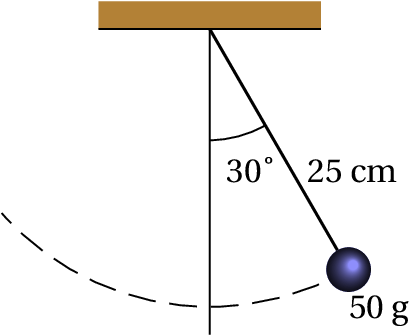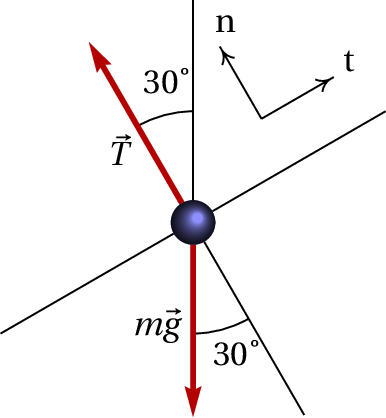Solution. It is convenient to make a free-body diagram for the sphere, that is, a diagram indicating only the external forces acting on the object. In this case, ignoring the air resistance, there are only two possible causes for these forces: the string and the gravitational attraction. Thus, the only external forces on the sphere are the tension of the string, which acts in the direction of the string and the weight, , in the vertical direction and downwards. The diagram shows those two forces and two angles which are equal.

Once the external forces are identified, a system of axes is chosen to calculate the components of those forces. In this case, since there is circular motion, it is convenient to use the tangential and normal axes, represented by the letters and in the diagram.

The normal axis points in the direction of the center of curvature of the trajectory, which in this case is the same direction of the string. The tangential axis is tangent to the circular trajectory; therefore, the velocity vector is perpendicular to the string. As the sphere moving higher, the velocity vector has the direction of the axis..

The tension on the string has only normal component. The tangential component of the weight is  N and the normal component is  N. Thus, the tangential and normal components of the total force are:

The tangential acceleration is hitherto unknown, but the normal acceleration can be obtained from the information given in the problem.

(SI units). Equating the tangential and normal components to and , The following system of equations is obtained:

The solution of that system is = -4.9 m/s2 and = 0.624 N. The negative sign of the tangential acceleration means that the speed of the sphere is decreasing.

## 4.3. Normal and frictional forces

When two surfaces are in contact there is a force among them, such as the forces , and in the example of the horse dragging a stone given in the previous section (figure 4.2). Those forces may point in different directions, but always in the sense from the surface towards the object on which that forces is acting.

It is convenient to separate the contact forces into two components: one component perpendicular to the surfaces in contact, called normal force and another component tangent to the surfaces, called frictional force.

The force of contact between two surfaces is actually distributed at various points on the surface. The resultant of all these forces can be represented as a single force at a point on the surface, separating the normal and tangential components (figure 4.5 ). The normal force, , points always in the sense that would make the two bodies in contact separate. The frictional force, , Can be in any sense of any of the directions tangent to the surface.Figure 4.5: normal force  N and frictional force  F f on a block on the surface of a table.

### 4.3.1. Static friction

When there is no relative movement between the two surfaces in contact, the frictional force is called static frictional force. It may be zero, or it may point in any direction tangent to the surfaces in contact.

In the example of the horse and the stone (figure 4.2), the tangential components of the contat forces on the horse's horseshoes, and , are static frictional forces. It is thanks toe the static frictional force with the road that wheeled vehicles can move and stop. It is also thanks to that force that we can walk: we push the ground with our feet and the reaction of the ground in the opposite direction makes us move forward.Figure 4.6: The force that allows the street car to climb a slope or go downhill with constant speed is the static frictional force between the wheels and the rails.

If the floor was covered with ice, our feet would slip making it harder for us to move or stop. This is because the magnitude of the static frictional force can not exceed a maximum value, which is proportional to the normal force:

(4.8)

where is a constant characteristic of the type of surfaces in contact, called coefficient of static friction. It usually has a value less than 1. In terms of the full contact force, this implies that the contact force is usually close to the normal direction, with a deviation of less than 45°.

Let us consider an example: the forces between the road and the tires of a bicycle. The frictional forces between the two tires and the road are both static frictional forces because the wheels do not slide. In the rear wheel the frictional force points forward in the direction the bicycle is moving (figure 4.7), and they are the reaction of the road to the action that the tire exerts on it in the opposite direction.

The static frictional force on the front wheel is in the direction opposite to the motion of the bicycle, because no traction is exerted by the rider on that wheel. The friction in the wheel axis and the rolling friction on the tire act against the rotation of that wheel, and the frictional force of the road allows the wheel to maintain its rotation in spite of those other forces.

If the speed of the bicycle is constant, the magnitude of the frictional force on the rear tire should be equal to the sum of the magnitudes of the frictional force on the front tire, the air resistance and the rolling friction upon the wheels.

### 4.3.2. Kinetic friction

When the two surfaces in contact slide between them, the frictional force is called kinetic frictional force. In the example of the horse and the stone (figure 4.2) the tangential component of the contact force acting on the stone is a kinetic frictional force.

The kinetic frictional force is always in the same direction of the velocity of the object relative to the surface, but in the opposite sense. Its magnitude is directly proportional to the normal force:

(4.9)

where is the coefficient of kinetic friction, which is usually less than the coefficient of static friction between the same surfaces.

Being in the opposite sense of the relative motion, the kinetic frictional force makes the relative velocity of the two surfaces decrease, but it can never reverse the direction of that relative velocity. At the instant that relative velocity becomes zero, the kinetic frictional force vanishes and it might be replaced by the static frictional force.

Thus, although the magnitude of the kinetic frictional force is constant, it depends implicitly on the velocity because it is always in the direction of the velocity and in the opposite sense. The vector expression of the kinetic frictional force is as follows:

(4.10)

Where is the velocity of the body on which the force acts, relative to the surface that produces it.

### Example 4.3

Compute the forces acting on the stone and the horse in figure 4.1, when they move with constant speed, the mass of the horse is 300 kg, the mass of the stone is 350 kg and the angle the rope does with the horizontal is 20°. The coefficient of kinetic friction between the stone and the ground is 0.4 and the coefficient of static friction between the horseshoes and the ground is 0.5.

Solution. The forces acting on the block and on the horse were shown in figure 4.2. Since the acceleration is zero, the sum of the horizontal and vertical components of the forces on the stone and the horse must be zero.

Starting with the stone, we can separate the force into the normal force , perpendicular to the ground, and the frictional force , tangent to the ground. The sum of the horizontal and vertical forces is,

Notice that the frictional force on the stone is due to kinetic friction, because the stone is sliding on the floor. Therefore, and replacing the values ​​of the coefficient of kinetic friction, mass of the stone and acceleration of gravity, we obtain a system of two equations with two unknowns

This system can be solved in Maxima with the following command:

(%i1) float(solve([T*cos(%pi/9)-0.4*N=0,N+T*sin(%pi/9)-3430=0]));
(%o1)

The normal force in the block is 2994 N and the tension in the rope is 1274 N.

Looking now at the free-body diagram for the horse (figure 4.2), the sums of the horizontal and vertical forces acting on the horse are:

It should be noted that in this case the relationship between the frictional forces and the normal forces is undetermined, because the friction is static. Replacing the value of the tension already obtained, the mass of the horse and the acceleration of gravity, we get the following system:

The sum of the normal forces on the horse's feet is 3376 N, and the sum of the frictional forces is 1198 N. In the chapter on dynamics of rigid bodies it will be explained how to determine the values ​​of and separately. For the time being, it follows only that the sum of these two forces is equal to 3376 N.

The values ​​of and can not be determined without additional information; it would be necessary to know the relation between the pressures that the horse is exerting on each foot, resulting from the action of different muscles on different joints. Since we are regarding the horse as a single rigid body, it is only possible for us to find the sum of those two forces.

The coefficient of static friction between the horseshoes and the road allows us to determine whether it is feasible for the horse to drag that stone, which is heavier than its own weight. The maximum static frictional force between the horseshoes and the floor is:

Since the sum of the two frictional forces and is less than this value, it is then possible for the horse to drag the stone. The horse could even drag a stone a bit heavier, but if the mass of the stone was bigger than a maximum value, the horseshoes would start to slip and the horse would not be able to drag the stone.

### 4.3.3. Fluid resistance

Most of the motions analyzed in this book are rigid body motions within fluids. In the example of the horse dragging a stone, the two bodies are moving into the air, which is a fluid. The air exerts a force of resistance, which is always opposite to the direction of motion.

In the two free-body diagrams in figure 4.2 the air resistance was ignored; it was assumed to be much smaller than the other forces, because the speed was low. Whe the speed is high, as it may happen for an object in free fall, the air resistance force is not negligible. In this section we discuss the dependence of that force on velocity.

The force that opposes the motion in fluids is produced mainly by two different mechanisms; the first one is related to the viscosity of the fluid and is due to the fact that the neighboring fluid layers are adhered to the body, moving with it and creating friction with other layers of fluid more distant, giving rise to a force directly proportional to the velocity.

The second mechanism has to do with the pressure difference generated in the fluid ahead and behind the moving object. The fluid is compressed in the front region and decompressed in the back. This difference of pressure produces a force opposite to the velocity and directly proportional to the square of its magnitude.

The two mechanisms are always present but in some conditions one of them may be much more appreciable than the other. The Reynolds number can be used as a measure of which of the two mechanisms is stronger; it is defined as

(4.11)

where Is a length of the order of magnitude of the straight section of the body seen in the direction of motion, is the speed of the body, is the density of the fluid and is the viscosity coefficient of the fluid. The Reynolds number has no units and it is not necessary to know its exact value but only its order of magnitude.

Stokes showed that when the Reynolds number is very low (order of magnitude of 1 or less), the fluid resistance force is proportional to the velocity. In the case of a sphere of radius , the expression for this force is:

(4.12)

When the Reynolds number is very high (order of magnitude of the thousands or more) the fluid resistance force is proportional to the square of the speed of the body:

(4.13)

where is the density of the fluid, is the drag coefficient of the body, smaller for sharp bodies and larger for less aerodynamic ones, and is the frontal area of the body, namely, the area of its projection in the direction of motion. In the case of a sphere of radius , its straight section is and the drag coefficient is approximately 1/2; therefore, the fluid resistance force on the sphere when the Reynolds number is high is:

(4.14)

If the velocity is very high, of the order of the speed of sound in the fluid (in air it is about 340 m/s) the fluid resistance force is proportional to the speed raised to an exponent greater than 2.

For a sphere of radius , the Reynolds number can be estimated by replacing by in equation 4.11. To decide which of the two equations, 4.12 or 4.14, is more accurate, one may start by admitting that the Reynolds number is low and solve the problem using equation 4.12. If the values ​​obtained lead to a low Reynolds number, it is then assumed that the solution is correct. Otherwise, one should solve the problem again using equation 4.14 and check that the result leads to a high Reynolds number but the velocity is less than the velocity of sound in that fluid (see problem 8 at the end of the chapter).

The resistance to motion of bodies in the air can be assumed to be proportional to the square of the velocity, unless the velocity is comparable to or greater than the speed of sound in the air (340 m/s). Indeed, the viscosity coefficient is 5 orders of magnitude smaller than the density, leading to high Reynolds numbers. The Reynolds number is only low if the velocity is too low, making the air resistance force small, or in microscopic bodies.

In the case of a free falling sphere in a fluid, the external forces acting on it are: the weight, , the upward buoyant force, which according to Archimedes' principal is equal to the weight of the fluid that the sphere displaces, , and the fluid resistance force. If the density of the sphere is greater than the density of the fluid, the weight is greater than the buoyant force and the sphere falls. In that case, the fluid resistance force points upwards and its magnitude is given by either expressions 4.12 or 4.14. When the free fall occurs in air, the resulting acceleration downward vertical acceleration is , where is a constant and . As shown in problem 12 of chapter 1, the speed reaches a limit value of .

## Questions

1. A book lies at rest on a table. Which of the following statements is correct:
1. There is no force acting on the book.
2. The book has no inertia.
3. There is no force acting on the table.
4. The book is in equilibrium.
5. The inertia of the book is equal to the inertia of the table.
2. Two metal balls are the same size but one weighs twice as much as the other. The two balls are dropped simultaneously, from the rest, from the top of a building. How do the times the two balls take to reach the ground compare?
1. The heavier ball takes about half the time of the lighter ball.
2. The lighter ball takes about half the time of the heaviest ball.
3. Both times are similar, but the heavier ball takes less time than the lighter one.
4. Both times are similar, but the lighter ball takes less time than the heavier one.
5. The two balls take exactly the same time.
3. A big truck collides frontally with a small car. What of the following states is true during the collision?
1. The truck exerts a greater force on the car than the force excerted by the car on the truck.
2. The car exerts a greater force on the truck than the force excerted by the truck on the car.
3. Neither one exerts force on the other. The car is crushed simply because it was in the path of the truck.
4. The truck exerts force on the car, but the car does not exert any force on the truck.
5. The truck exerts a force on the car and the car exerts the same force on the truck.
4. A stone is thrown vertically upwards. At the highest point of the trajectory of the stone:
1. Its velocity and acceleration point down.
2. Its velocity points upwards and its acceleration points downwards.
3. Its velocity ​​and acceleration are both zero.
4. Its velocity is zero and its acceleration points down.
5. Its velocity points downward and its acceleration is zero.
5. A woman pushes a large box with a constant horizontal force. The force exerted by the woman causes the box to move horizontally with constant speed . We can then conclude that the magnitude of force exerted by the woman:
1. Is equal to the weight of the box.
2. Is larger than the weight of the box.
3. Is equal to the total force that opposes the movement of the box.
4. Is larger than the total force that opposes the movement of the box.
5. Is larger than the weight and the total force that opposes the movement of the box.

## Problems

1. A 70 kg person climbs to the sixth floor of a building using an elevator. The elevator starts from rest on the ground floor, accelerates until the second floor with uniform acceleration of 2 m/s2, keeps the speed constant between the second and fourth floors and slows down between the fourth and sixth floors with uniform acceleration of −2 m/s2. Determine the magnitude of the normal force in the person's feet in each part of the climb.
2. A block of mass 30 kg is on a horizontal surface with coefficient of kinetic friction equal to 0.35. There is an external force of 100 N acting on the block, which makes an angle of 30° with the horizontal. Determine the value of the acceleration of the block.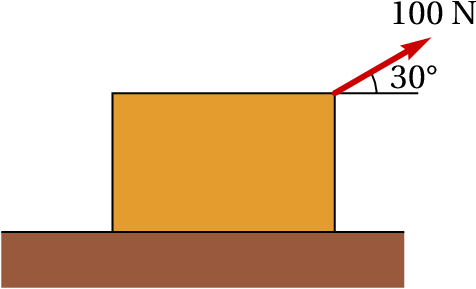3. A block of mass  Kg slides down the surface of an incline with 4 m of base and 3 m of height. If the coefficient of kinetic friction between the block and the surface of the incline is 0.25, compute the value of the frictional force on the block.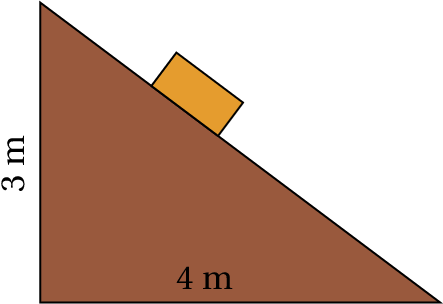4. An object with mass of 2 kg moves with initial velocity  m/s, when an external force (SI units) is applied during 5 seconds. Determine:
1. The final velocity after 5 seconds.
2. The impulse given by the external force during the 5 seconds.
5. A 72 kg man pushes an 8 kg wooden box on a horizontal floor, exerting a horizontal force on it that makes it slide on the floor. On the box is placed a book with 0.6 kg. The man, the box and the book move together, with acceleration equal to 0.5 m/s2. Determine the values ​​of the frictional forces between the floor and the box, between the box and the book and between the floor and the feet of the man, ignoring the air resistance and knowing that the coefficients of static friction ( ) and kinetic friction ( ) are: between the floor and the box, and ; between the box and the book, and ; between the ground and the feet of the man, and .
6. A 1230 kg car climbs an 8 percent slope with constant speed. Determine:
1. The value of the total frictional force (sum of the forces on the four tires).
2. The minimum value of the coefficient of static friction between the road and the tires so that the car can climb up the ramp.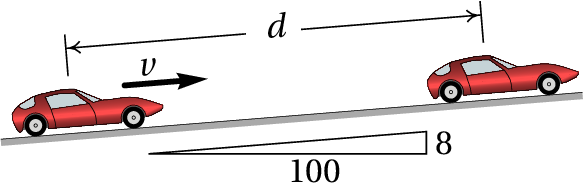7. To determine the rigidity of a material, a block of that material is place 30 cm below a metal cone of 0.3 kg. The cone is allowed to fall freely from rest then penetrating the block until it stops after moving a distance inside it. While the cone is penetrating, the block exerts on it a force opposite to its velocity directly proportional to the square of the penetrated distance; that is, the magnitude of the force is , where is the distance penetrated by the tip of the cone and is a positive constant that measures the rigidity of the material. If the maximum distance that the cone penetrates is  cm, determine the value of the rigidity constant for this material.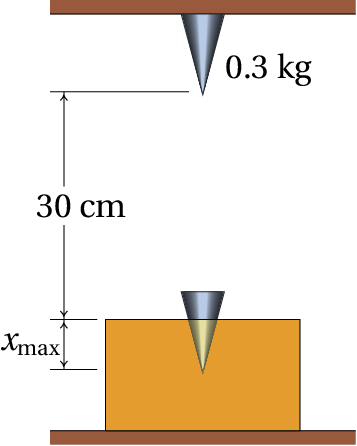8. A sphere of radius and density falls freely inside a fluid with a density and viscosity coefficient . (a) Find the expressions for the terminal velocity when the resistance of the fluid is proportional to the velocity and when it is proportional to the square of the velocity. (b) Compute the terminal velocities of a sphere of diameter 1 cm made of steel, with density 7800 kg/m3, inside glycerin, water and air. In each case determine the value of the Reynolds number. Use the data in the following table:
 Fluid Viscosity (kg/(m·s)) Density (kg/m3) Glycerin 1.5 1200 Water 10−3 1000 Air 1.8×10−5 1.2
1. A raindrop with radius of 1 mm (use 1000 kg/m3 for the density of water).
2. A hail stone with a radius of 1 cm (the density of the ice is 917 kg/m3).
3. A table tennis ball with radius of 1.9 cm and mass of 0.0024 kg.
4. A tennis ball with radius of 3.25 cm and mass of 0.062 kg. (Use the results of the previous problem).
10. To measure the coefficient of static friction between a block and a disc, the disc was rotated with constant angular acceleration rad/s2. The disc starts from rest at and at  s the block begins to skid over the disk. Determine the value of the coefficient of static friction.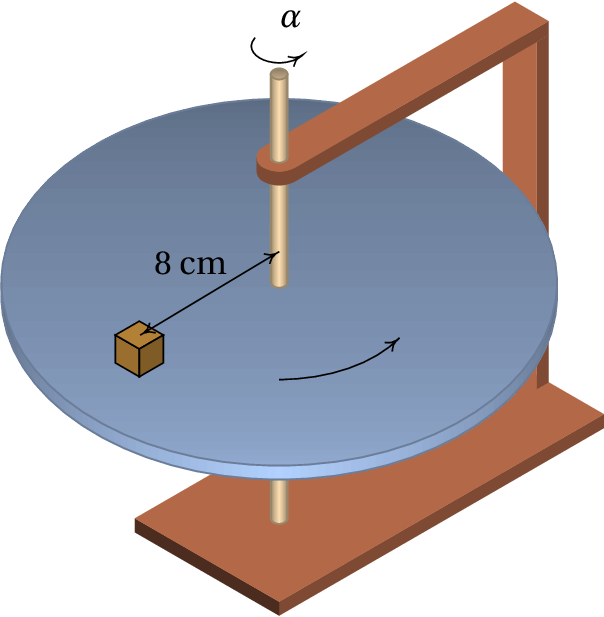11. A ball with mass 0.8 kg is initially kept at rest by two wires, as shown in the figure. The wire on the left is suddenly cut off. Determine the tension on the right-hand wire before the other wire was cut and the exact instant after the wire is cut (assume that the wire weights are negligible).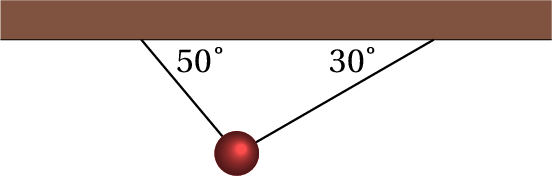Questions: 1. D. 2. C. 3. E. 4. D. D. 5. C.

Problems

1. Between the gound level and the 2nd floor, 826 N. Between the 2nd and 4th floors, 686 N. Between the 4th and 6th floors, 546 N.
2. 0.040 m/s2
3. 4.12 N.
4. (a) ( ) m/s. (b ) ( ) N·s.
5. The frictional force between the box and the book is 0.3 N, the frictional force between the box and the floor is 16.856 N, and the frictional force between the floor and the man's feet is 57.156 N.
6. (a) 961.2 N. (b) 0.08.
7. 24 696 N / m 2 .
8. ( a ) With resistance proportional to the speed: .
With resistance proportional to : .
(b) In glycerin: m/s, ; in water: m/s, ; in air: m/s, .
9. (a) 6.60 m/s = 23.7 km/h. (b) 20.0 m/s = 71.9 km/h. (c) 8.25 m/s = 29.7 km/h. (d) 24.7 m/s = 88.8 km/h.
10. 0.143
11. Before: N. after: N.
Wrong

(Click to continue)

Wrong

(Click to continue)

Wrong

(Click to continue)

Correct

(Click to continue)

Wrong

(Click to continue)

Wrong

(Click to continue)

Wrong

(Click to continue)

Correct

(Click to continue)

Wrong

(Click to continue)

Wrong

(Click to continue)

Wrong

(Click to continue)

Wrong

(Click to continue)

Wrong

(Click to continue)

Wrong

(Click to continue)

Correct

(Click to continue)

Wrong

(Click to continue)

Wrong

(Click to continue)

Wrong

(Click to continue)

Correct

(Click to continue)

Wrong

(Click to continue)

Wrong

(Click to continue)

Wrong

(Click to continue)

Correct

(Click to continue)

Wrong

(Click to continue)

Wrong

(Click to continue)